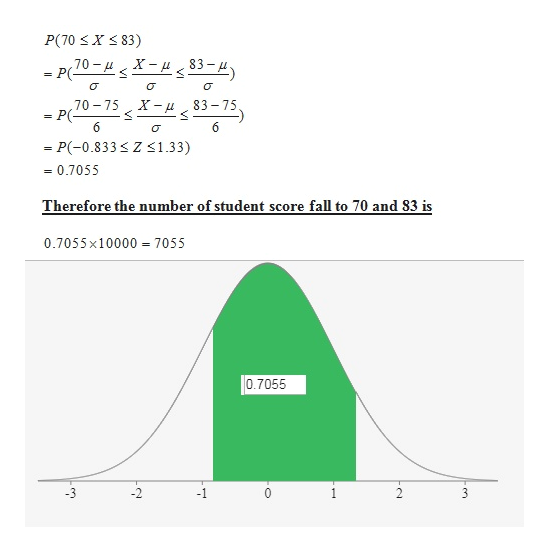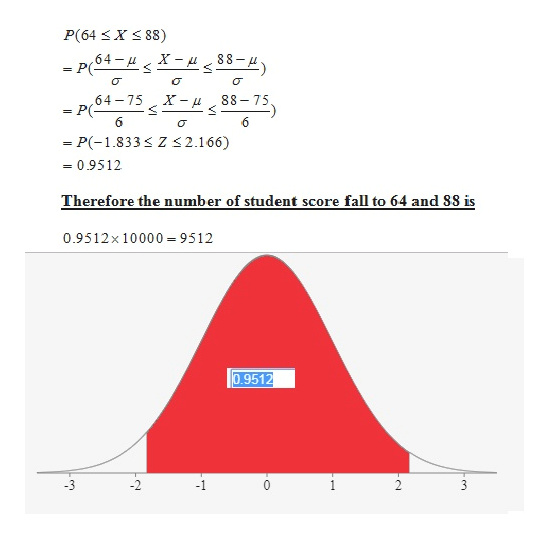# Midterm exam scores are normally distributed with a mean of 75 and a standard deviation of 6. If the exam is given to 10,000 students, we would expectA. Almost all scores to fall between 70 and 83.B. Half the scores to fall between 64 and 88.C. No one to have received a score of 87.D. No one to have received a score below 63.E. Just over one third of the scores to fall between 75 and 81

Question
78 views

Midterm exam scores are normally distributed with a mean of 75 and a standard deviation of 6. If the exam is given to 10,000 students, we would expect

• A. Almost all scores to fall between 70 and 83.
• B. Half the scores to fall between 64 and 88.
• C. No one to have received a score of 87.
• D. No one to have received a score below 63.
• E. Just over one third of the scores to fall between 75 and 81
check_circle

Step 1

According to the question , Midterm exam scores are normally distributed with a mean of 75 and a standard deviation of 6. If the exam is given to 10,000 students.

We define X be the random variable normally distributed with mean 75 and standard deviation 6 shows the marks of each student.

Therefore:

A: Almost all scores to fall between 70 and 83.

This statement is not true as only 7055 number of students fall to 70 and 83.

Explanation is follows:help_outlineImage TranscriptioncloseP(70 X 83) 70- X- 83- 83 75 X- 70 75 P( - 6 = P(-0.833Z1.33) 0.7055 Therefore the number of student score fall to 70 and S3 is 0.7055x10000 = 7055 0.7055 2 en fullscreen
Step 2

B. Half the scores to fall between 64 and 88.

This statement also not true as more than half  (9512) of the students get 64 and 88 marks .

Explanation as follows:help_outlineImage TranscriptioncloseP(64 88) 88-H X 64 u P( = X-u 64 = P(= 6 75 88 75 - P(-1.833 Z <2.166) 0.9512 Therefore the numb er of student score fall to 64 and 88 is 0.9512x 10000 9512 0.9512 fullscreen
Step 3

C. No one to have received a score of 87.

As the X variable is normally distrubuted with mean 75 and standard deviation 6 ...

### Want to see the full answer?

See Solution

#### Want to see this answer and more?

Solutions are written by subject experts who are available 24/7. Questions are typically answered within 1 hour.*

See Solution
*Response times may vary by subject and question.
Tagged in

### Statistics